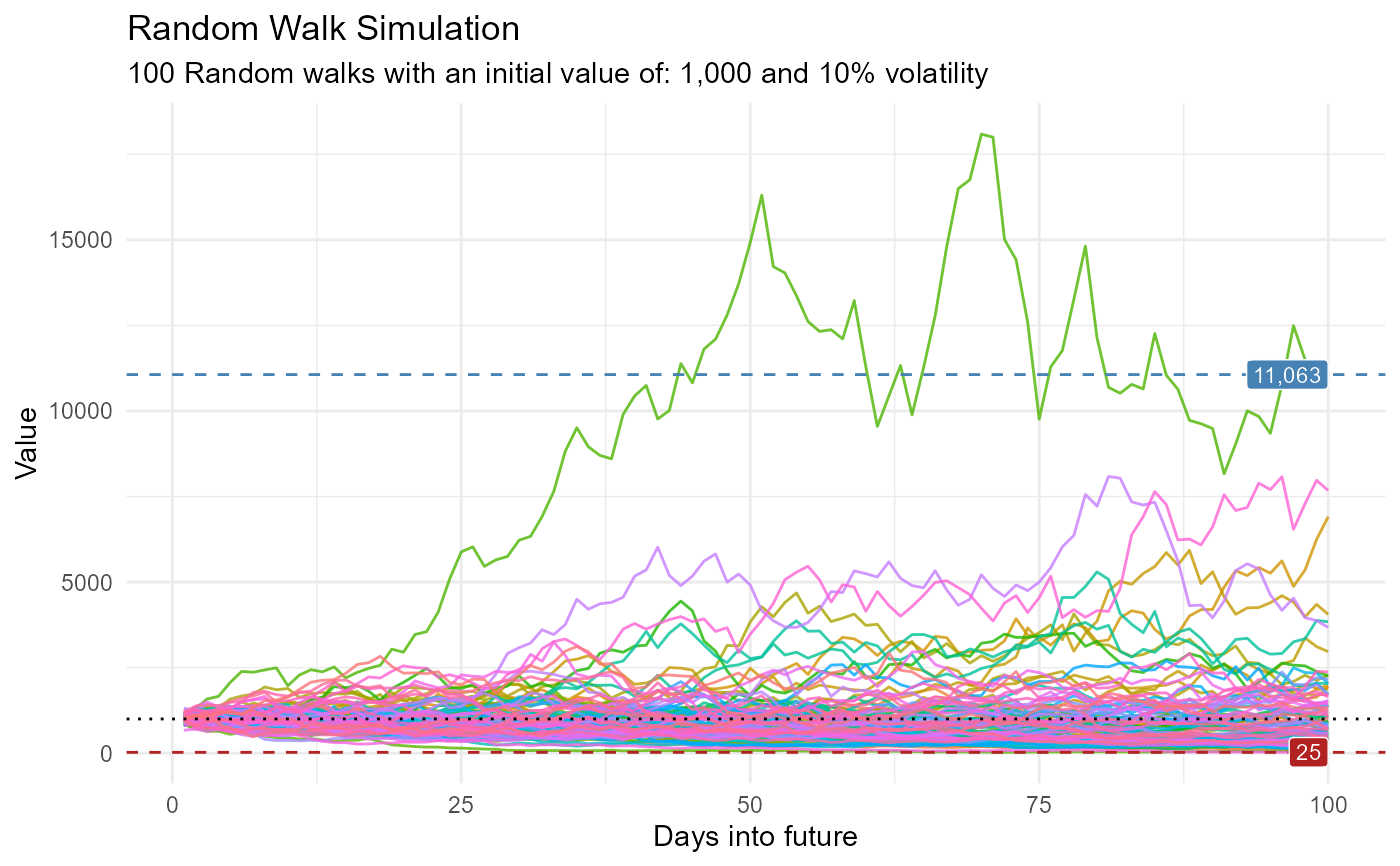Get layers to add to a ggplot graph from the ts_random_walk() function.

## Usage

ts_random_walk_ggplot_layers(.data)

## Arguments

.data

The data passed to the function.

## Value

A ggplot2 layers object

## Details

• Set the intercept of the initial value from the random walk

• Set the max and min of the cumulative sum of the random walks

## Author

Steven P. Sanderson II, MPH

## Examples

library(ggplot2)

df <- ts_random_walk()

df %>%
ggplot(
mapping = aes(
x = x
, y = cum_y
, color = factor(run)
, group = factor(run)
)
) +
geom_line(alpha = 0.8) +
ts_random_walk_ggplot_layers(df)